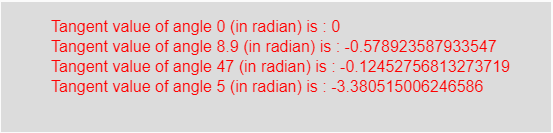# p5.js | tan() function

The tan() function in p5.js is used to calculate the tangent value of an angle in radians taken as the input parameter of the function.

Syntax:

`tan(angle)`

Parameters: This function accepts a single parameter angle which is an angle in radian whose tangent value is to be calculated.

Return Value: It returns the tangent value of an angle in radian taken as the input parameter.

Below program illustrates the tan() function in p5.js:

Example: This example uses tan() function to get tangent value of an angle in radian.

 `function` `setup() {  ` `  `  `    ``// Create Canvas of given size  ` `    ``createCanvas(550, 130);  ` `}  ` `  `  `function` `draw() {  ` `      `  `    ``// Set the background color  ` `    ``background(220);  ` `      `  `    ``// Initialize the parameter with ` `    ``// angles in radian only ` `    ``let a = 0;  ` `    ``let b = 8.9;  ` `    ``let c = 47; ` `    ``let d = 5; ` `      `  `    ``// Call to tan() function  ` `    ``let v = tan(a); ` `    ``let w = tan(b); ` `    ``let x = tan(c); ` `    ``let y = tan(d); ` `      `  `    ``// Set the size of text  ` `    ``textSize(16);  ` `      `  `    ``// Set the text color  ` `    ``fill(color(``'red'``));  ` `    `  `    ``// Getting tangent value  ` `    ``text(``"Tangent value of angle 0 (in radian) is : "` `+ v, 50, 30); ` `    ``text(``"Tangent value of angle 8.9 (in radian) is : "` `+ w, 50, 50); ` `    ``text(``"Tangent value of angle 47 (in radian) is : "` `+ x, 50, 70); ` `    ``text(``"Tangent value of angle 5 (in radian) is : "` `+ y, 50, 90);      ` `}  `

Output:Note: In the above code, the input angle should be in radian. For converting the degree into radian use the following formula:

`Angles_in_radian = (π/180)*angles_in_degree`

Reference: https://p5js.org/reference/#/p5/tanMy Personal Notes arrow_drop_upCheck out this Author's contributed articles.

If you like GeeksforGeeks and would like to contribute, you can also write an article using contribute.geeksforgeeks.org or mail your article to contribute@geeksforgeeks.org. See your article appearing on the GeeksforGeeks main page and help other Geeks.

Please Improve this article if you find anything incorrect by clicking on the "Improve Article" button below.

Article Tags :

Be the First to upvote.

Please write to us at contribute@geeksforgeeks.org to report any issue with the above content.